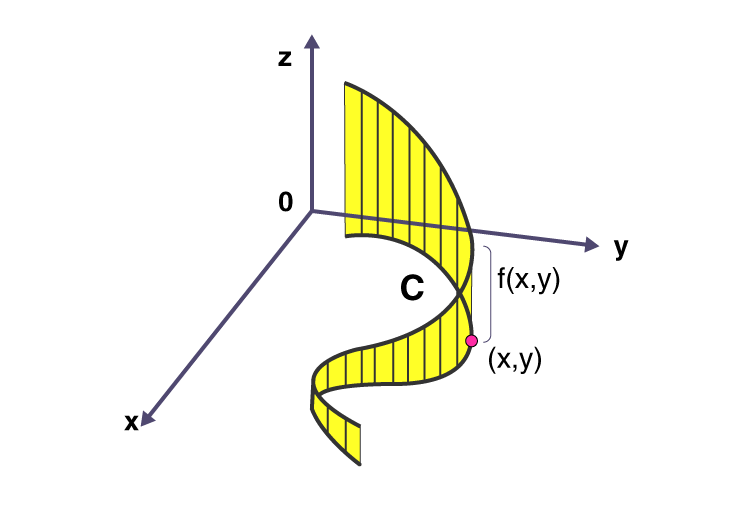Jet Set Go! All about Aeroplanes Jet Set Go! All about Aeroplanes

# Line Integral

In Calculus, a line integral is an integral in which the function to be integrated is evaluated along a curve. A line integral is also called the path integral or a curve integral or a curvilinear integral. In this article, we are going to discuss the definition of the line integral, formulas, examples, and the application of line integrals in real life.

## Line Integral Definition

A line integral is integral in which the function to be integrated is determined along a curve in the coordinate system. The function which is to be integrated may be either a scalar field or a vector field. We can integrate a scalar-valued function or vector-valued function along a curve. The value of the line integral can be evaluated by adding all the values of points on the vector field.## Line Integral Formula

The line integral for the scalar field and vector field formulas are given below:

Line integral Formula for Scalar Field

For a scalar field with function f: U ⊆ Rn → R, a line integral along with a smooth curve, C ⊂ U is defined as:

$$\begin{array}{l}∫_C f(r) ds = \int_{a}^{b} f[r(t)] |r’(t)| dt\end{array}$$

Here, r: [a, b]→C is an arbitrary bijective parametrization of the curve.

r (a) and r(b) gives the endpoints of C and a < b.

Line integral Formula for Vector Field

For a vector field with function, F: U ⊆ Rn → Rn, a line integral along with a smooth curve C ⊂ U, in the direction “r” is defined as:

$$\begin{array}{l}∫_C F(r). dr =\int_{a}^{b} F[r(t)] . r’(t)dt.\end{array}$$

Here, “.” represents the dot product.

## Application of Line Integral

Line integral has several applications. A line integral is used to calculate the surface area in the three-dimensional planes. Some of the applications of line integrals in the vector calculus are as follows:

• A line integral is used to calculate the mass of wire.
• It helps to calculate the moment of inertia and centre of mass of wire.
• It is used in Ampere’s Law to compute the magnetic field around a conductor.
• In Faraday’s Law of Magnetic Induction, a line integral helps to determine the voltage generated in a loop.
• Line integral helps to calculate the work done by a force on a moving object in a vector field.

### Line Integral Example

Go through the line integral example given below:

Example: Evaluate the line integral ∫C F. dr where F(x, y, z) = [P(x, y, z), Q(x, y, z), R(x, y, z)] = (z, x, y), and C is defined by the parametric equations, x = t2, y = t3 and z = t2 , 0 ≤ t ≤ 1.

Solution:

Given that, the function, F(x, y, z) = [P(x, y, z), Q(x, y, z), R(x, y, z)] = (z, x, y)

Parametric equations: x = t2, y = t3 and z = t2 , 0 ≤ t ≤ 1.

We know that,

C F. dr = ∫C P dx + Q dy + R dz

C F. dr = 01 z(t) x’(t)dt + x(t) y’(t)dt + y(t) z’(t)dt

= 01 t2 (2t)dt + t2 (3t2)dt + t3 (2t) dt

= 01 2t3 dt + 3t4 dt + 2t4dt

= 01 (5t4 + 2t3) dt

$$\begin{array}{l}= \left ( 5\frac{t^{5}}{5}+2\frac{t^{4}}{4} \right )_{0}^{1}\end{array}$$

Substitute the limits, we get,

C F. dr = 3/2

Therefore, the line integral for the given function is 3/2.

Keep visiting BYJU’S – The Learning app for more Maths related articles and download the app to get the interactive videos.